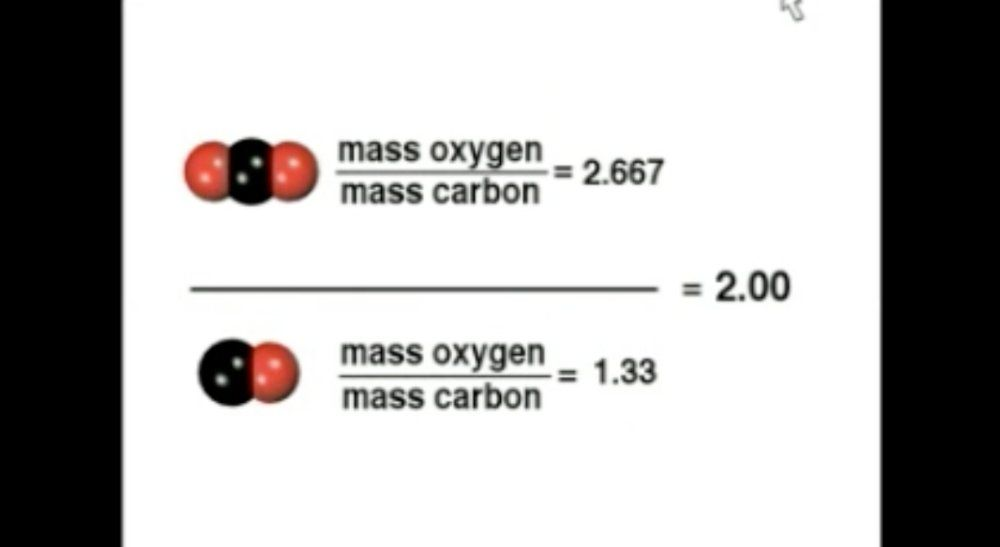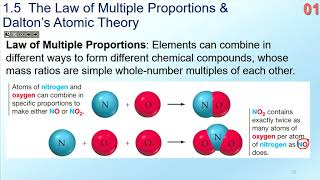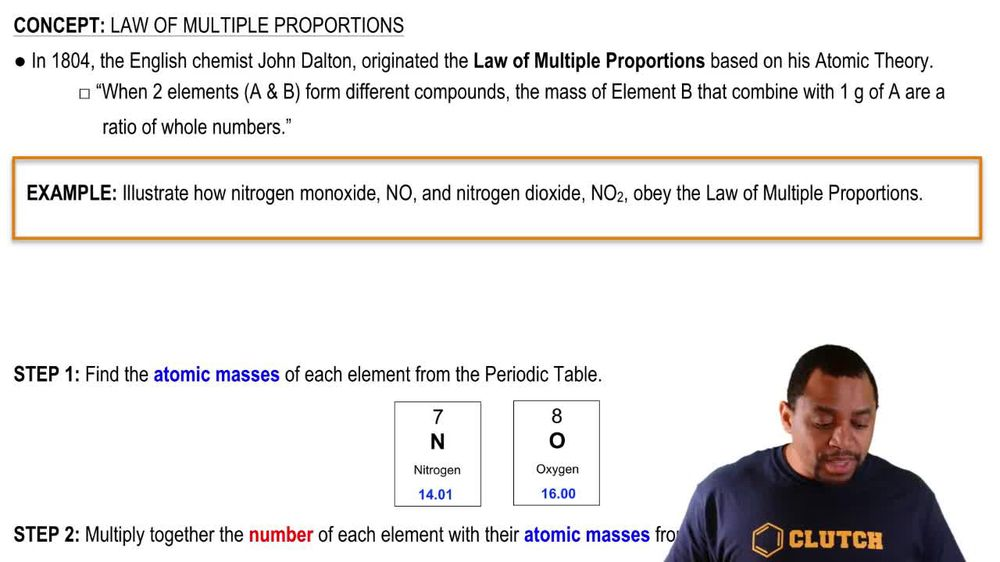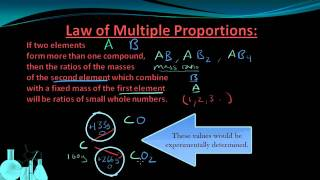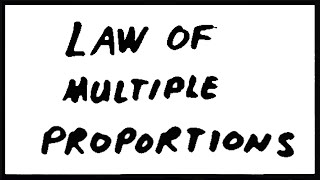Start typing, then use the up and down arrows to select an option from the list.
1. 2. Atoms & Elements2. Law of Multiple Proportions
Problem

# In a series of experiments, a chemist prepared three different compounds that contain only iodine and fluorine and determined the mass of each element in each compound: Compound Mass of Iodine (g) Mass of Fluorine (g) 1 4.75 3.56 2 7.64 3.43 3 9.41 9.86 (a) Calculate the mass of fluorine per gram of iodine in Compound 2.

Relevant Solution1m
Play a video:
Hey everyone in this example we're told that a compound containing only xenon and florian was analyzed and the mass of each element in the sample was found to be 0.8803 g of xenon and 0.697 g of flooring. We need to give the mass of flooring program of xenon in the compound so we want to recall our formula for the mass ratio. And this is going to be found by taking our mass of flooring which is our smaller mass. And dividing it by our mass of xenon which had the larger mass given in the prompt. So what we would find is that our mass ratio is going to equal a value of in our numerator for our massive floor. In the prompt gives us as 0.697 g of flooring in our denominator. We're going to have our mass of xenon given in the prompt as 0.803 g of xenon. And what this quotient gives us is a mass ratio equal to a value of 0.868 g of flooring per gram of xenon. And this will be our final answer to complete this example. So I hope that everything I ran through is clear. If you have any questions, please leave them down below and I will see everyone in the next practice video